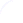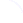Search
 Submission Procedure share: |Follow usavailable in:   PDF (206 kB) PS (226 kB)

get:
 Similar Docs BibTeX Write a comment
get:
 Links into Future

### Numerical Treatment of a Data Completion Problem in Heat Conduction Modelling

Augusto C. de Castro Barbosa (UERJ - Rio de Janeiro State University, Brazil)

Carlos A. de Moura (UERJ - Rio de Janeiro State University, Brazil)

Jhoab P. de Negreiros (UNIGRANRIO - Great Rio University, Brazil)

J. Mesquita de Souza Aguiar (UERJ - Rio de Janeiro State University, Brazil)

Abstract: This work deals with a question in the mathematical modelling for the temperature evolution in a bar, for a long time linked as an inverse problem. The onedimensional model is the parabolic partial differential equation ut = α uxx, known as the heat diffusion equation. The classic direct problem (DP) involves this equation coupled to a set of constraints: initial and boundary conditions, in such a way as to guarantee existence of a unique solution. The data completion (DC) problem hereby considered may be described as follows: the temperature at one of the bar extreme points is unknown but there is a fixed interior point where it may be measured, for all time. Finite difference algorithms (FDA) were tested to approximate the solution for such a problem. The important point to be emphasized is that FDA may show up distinct performances when applied to either DP or DC, which is due to the way the discrete variables follow up the mesh steps - advancing in time, for the first case, on the space direction, for the other.

Keywords: data completion, diffusion equation, finite differences, heat conduction,, inverse problem

Categories: G.1.8, J.2, J.6